U.S. Department of Transportation
1200 New Jersey Avenue, SE
Washington, DC 20590
202-366-4000

Federal Highway Administration Research and Technology
Coordinating, Developing, and Delivering Highway Transportation InnovationsThis report is an archived publication and may contain dated technical, contact, and link information
 Federal Highway Administration > Publications > Research Publications > 04046 > 10.Cfm > Evaluation of Procedures for Quality Assurance Specifications
 Publication Number: FHWA-HRT-04-046 Date: October 2004

# 10. The Composit Payment Factor

Most specifications employ multiple quality characteristics for acceptance and payment. Therefore, one issue that must be addressed is how to combine these multiple characteristics to come up with a single payment factor for a lot. In chapter 8, a quality measure (PWL) was selected for use in an equation to determine payment for a single quality characteristic. A number of different methods for determining composite payment factors are currently in use. These can be referred to as traditional methods for determining a composite payment factor.

Through the years, various agencies have considered at least five different approaches for combining a number of payment factors for individual acceptance quality characteristics into a single composite payment factor. These approaches include:

• Averaging (possibly with weighting factors) the individual payment factors.
• Multiplying the individual payment factors.
• Summing the individual payment adjustments.
• Using the maximum individual payment factor.
• Using the minimum individual payment factor.

The approach of using the maximum individual payment factor could be thought of as allowing the contractor the benefit of the doubt. The approach using the minimum individual payment factor as the composite payment factor is based on the weak link theory (i.e., the lowest payment factor indicates the value for all of the quality characteristics). For the other three approaches, the concept is that all of the individual factors contribute to the total. However, the composite payment for the three approaches can be quite different depending on the value of the individual payment factors. All of these approaches for determining a composite payment factor were analyzed by computer simulation.

### ANALYSES PROCEDURES

To simplify the simulation process and to allow for graphical presentation of the results, the analyses of the different methods for determining a composite payment factor were conducted only for the case where there are two individual, but possibly correlated, payment characteristics and where PWL was used for the quality measure. Only the case where the two individual quality characteristic populations have the same PWL value is presented here. All analyses were conducted using a sample size = 5, although similar trends would be expected for other sample sizes; however, the variability would be greater for smaller sample sizes and less for larger sample sizes.

The methods considered for combining the individual payment factors were:

• Averaging the individual payment factors ((0.5 X P1) + (0.5 X P2)).
• Using a weighted average of the individual payment factors ((0.75 X P1) + (0.25 X P2)).
• Multiplying the individual payment factors (P1 X P2).
• Summing the individual payment adjustments (P1 + P2 - 100).
• Using the maximum individual payment factor (Max(P1, P2)).
• Using the minimum individual payment factor (Min(P1, P2)).

Since the two simulated populations had equal PWL values, it was anticipated that the average and weighted average methods would have similar results for the EP and would exhibit similar trends for standard deviation, although the magnitudes of the standard deviation values would be different because of the different weighting factor multipliers used.

Each of the above payment calculation methods was simulated for three different equal population PWL values (90, 70, and 50). Each of the PWL values was simulated with using correlation coefficients ranging from -1.0 to +1.0. The results of these analyses are presented below.

### ANALYSES RESULTS

The results of the analyses are shown in figures 87 through 95. Figures 87 through 92 show the expected combined payment and standard deviation of the individual combined payments for correlation coefficients = -1.00, -0.90, -0.75, -0.50, -0.25, -0.10, 0.00, +0.10, +0.25, +0.50, +0.75, +0.90, and +1.00 for each of the six methods identified above. The simulation results for three different PWL values (90, 70, and 50) are included in each figure.

Figures 87 through 90 show similar trends regarding EP and standard deviation values for the simulated combined payments using the averaging, weighted average, multiplying, and summing methods. These figures show that the level of correlation between the two acceptance variables does not affect the EP values for these four methods. These figures also show that there is a similar effect on the standard deviation of the payment values for both different PWL values and for different levels of correlation. For the 50 and 70 PWL values, there is a consistently increasing trend in standard deviation as the correlation coefficient goes from -1.0 to +1.0. However, for the 90 PWL value, the standard deviations decrease slightly as the correlation coefficients approach 0.0 from both the positive and negative directions.

The reason for these different trends in the standard deviation results rests in the fact that the upper limit of 100 PWL (you cannot have more than 100 PWL) is reached much more often for a population of 90 PWL. This boundary has the effect of limiting the spread of the individual payment values, thereby reducing the standard deviation values. The discussions in chapter 8 that explained why the standard deviation was smaller for negative correlations and larger for positive correlations explain this similar trend in figures 87 through 90.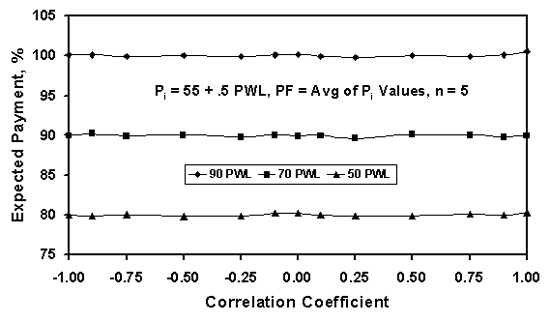Figure 87a. Simulation results of expected payment for the averaging method, combining two populations with equal PWL values.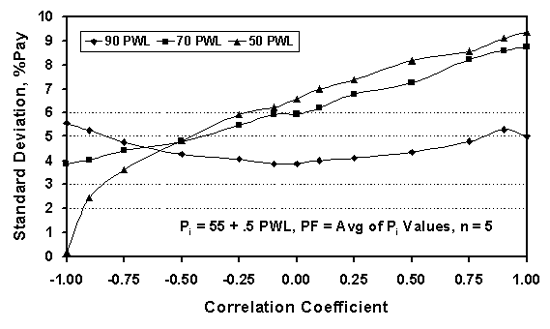Figure 87b. Simulation results of standard deviation values for the averaging method, combining two populations with equal PWL values.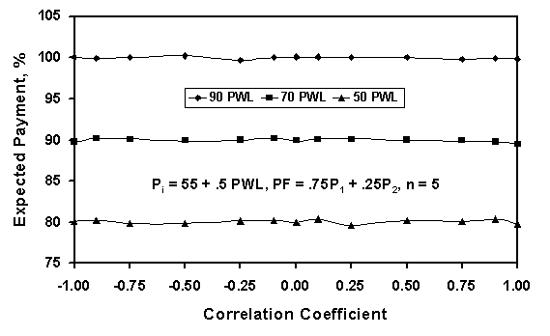Figure 88a. Simulation results of expected payment for the weighted average method, combining two populations with equal PWL values.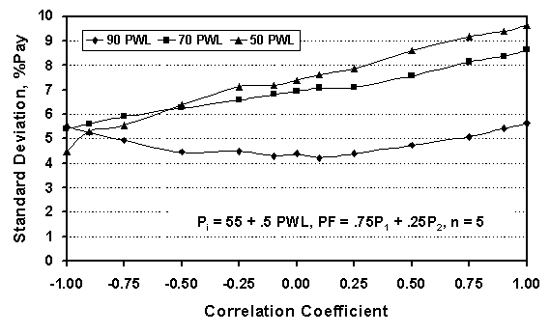Figure 88b. Simulation results of standard deviation values for the weighted average method, combining two populations with equal PWL values.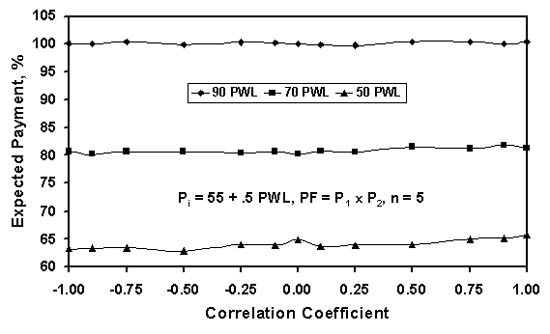Figure 89a. Simulation results of expected payment for the multiplication method, combing two populations with equal PWL values.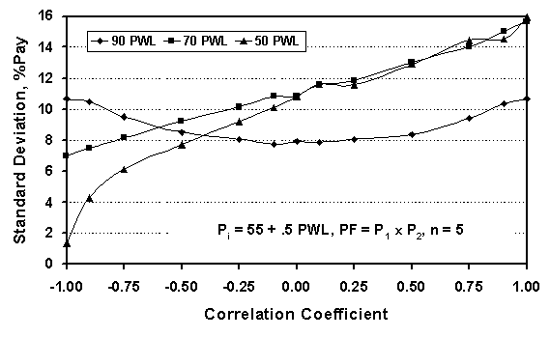Figure 89b. Simulation results of standard deviation values for the multiplication method, combining two populations with equal PWL values.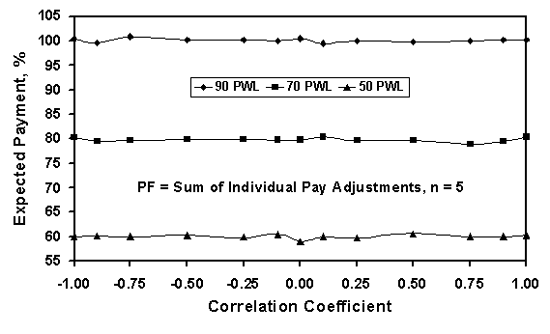Figure 90a. Simulation results of expected payment for the summation method, combining two populations with equal PWL values.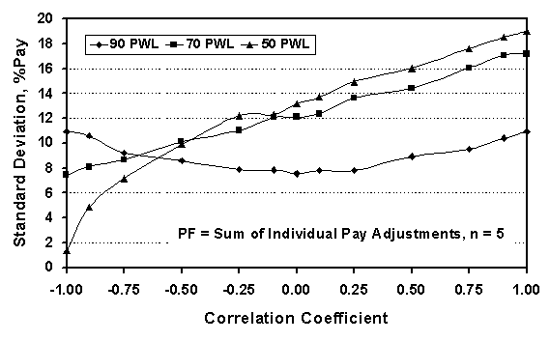Figure 90b. Simulation results of standard deviation values for the summation method, combining two populations with equal PWL values.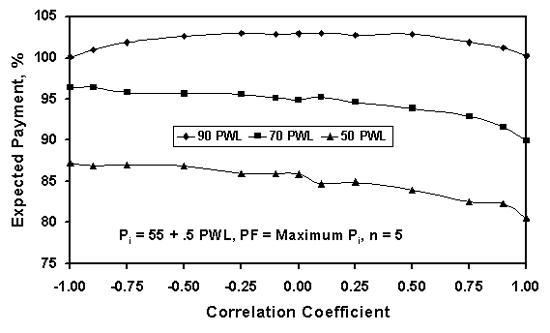91a. Simulation results of expected payment for the maximum method, combining two populations with equal PWL values.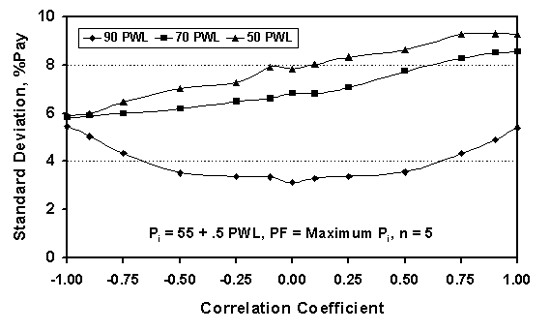Figure 91b. Simulation results of standard deviation values for the maximum method, combining two populations with equal PWL values.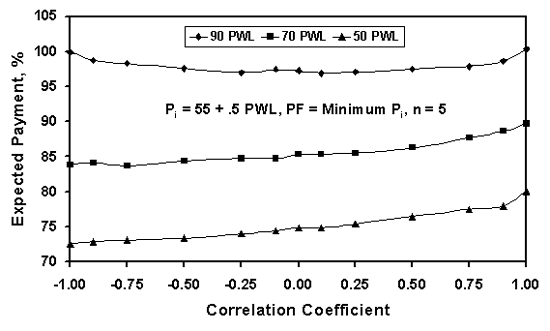Figure 92a. Simulation results of expected payment for the minimum method, combining two populations with equal PWL values.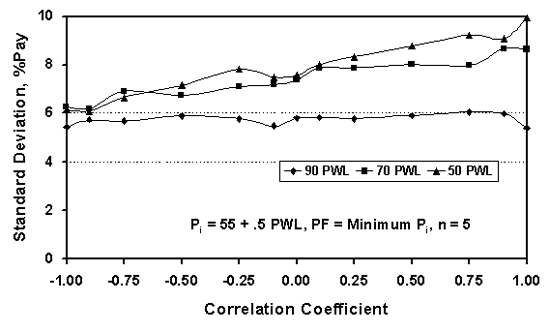Figure 92b. Simulation results of standard deviation values for the minimum method, combining two populations with equal PWL values.

Somewhat different trends are shown in figures 91 and 92, which show the results for the maximum and minimum methods, respectively, for determining the combined payment factor. For these methods, for populations of 70 and 50 PWL, the EP values vary with the level of correlation between the two acceptance variables. This is not a desirable situation. In the first four methods considered, the EP for each level of quality, as indicated by the PWL, was independent of the correlation coefficient. For these methods, the variability was affected by the correlation value, but the EP was centered on the correct value.

In figure 91, not only does the variability increase as the correlation goes from -1.0 to +1.0, but the EP values for populations of 70 and 50 PWL decrease over this same range. In figure 92, the EP values for populations of 70 and 50 PWL increase as the correlation goes from -1.0 to +1.0. For the maximum and minimum methods, the variability increases and the increasing or decreasing EPs are related to one another. If the average value remains constant, but variability increases, then there is more spread about the average. Since it is the single largest value that establishes the payment for the maximum method, the increased variability means that the maximum values will, on average, be higher. Similarly, for the minimum method, the increased variability also means that the minimum values will be lower.

#### Comparing the Methods

Figures 93 through 95 show the same results as figures 87 through 92, but plotted by the actual PWL values rather than the payment combination method. In these figures, it is clear that the EP values for the averaging, weighted average, multiplying, and summing methods do not vary with the correlation between the acceptance variables, and that the maximum and minimum methods do vary.

When the EP values vary with the correlation coefficient, it means that the average payment values will be different in the long run for different correlation relationships between the two acceptance variables. When the EP values do not vary with the correlation coefficient, but the standard deviation does vary, this means that even though the average payment values in the long run will be the same, there will be a difference in the spread or range of payment values that may be estimated for individual lots.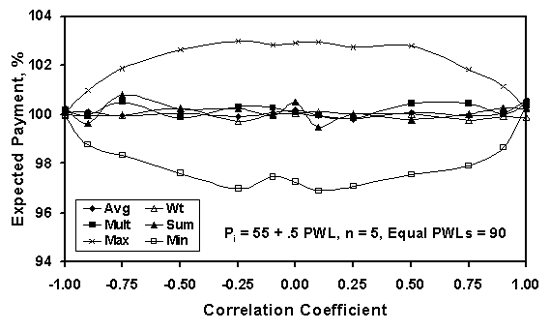Figure 93a. Comparison of simulation results for various methods for combining individual expected payment factors for two populations with PWL = 90.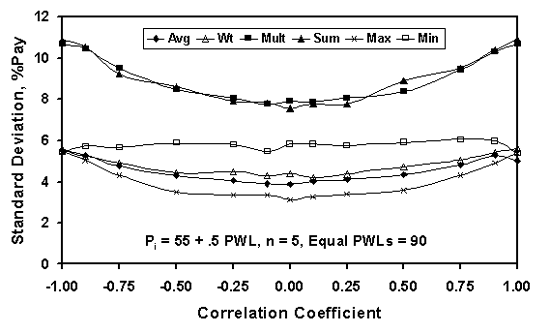Figure 93b. Comparison of simulation results for various methods for combining individual standard deviation payment factors for two populations with PWL = 90.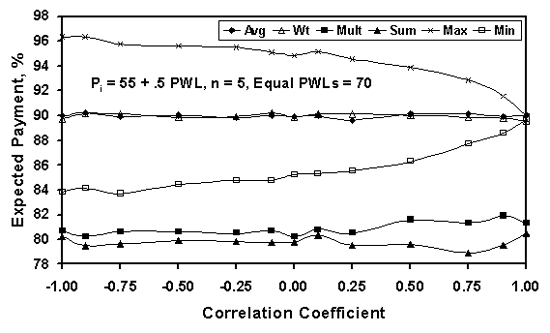Figure 94a. Comparison of simulation results for various methods for combining individual expected payment factors for two populations with PWL = 70.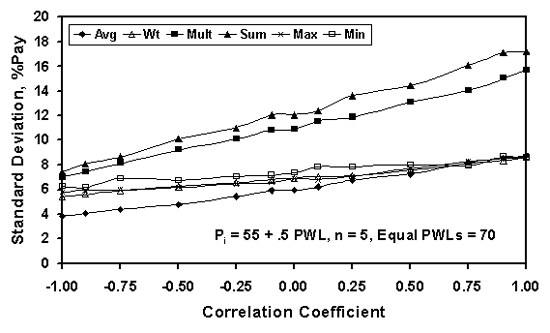Figure 94b. Comparison of simulation results for various methods for combining individual standard deviation payment factors for two populations with PWL = 70.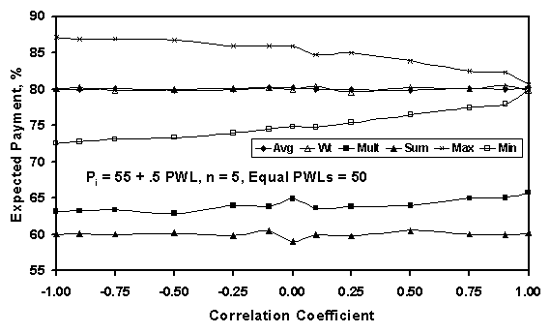Figure 95a. Comparison of simulation results for various methods for combining individual expected payment factors for two populations with PWL = 50.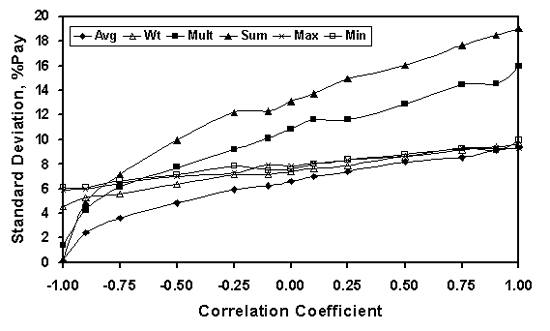Figure 95b. Comparison of simulation results for various methods for combining individual standard deviation payment factors for two populations with PWL = 50.

### CONCLUSION: TRADITIONAL COMPOSITE QUALITY MEASURES

The maximum and minimum methods essentially disregard the quality characteristics that do not give the maximum or minimum payment value, respectively. This seems to ignore useful information from the other quality characteristics. The averaging, multiplying, and summing methods for combining individual payment factors implicitly assume that each individual payment property is equally important. This is probably, but not always, a valid assumption.

Weighted average composite payment factors are intuitively appealing since it is very likely that all payment quality characteristics do not have an identical impact on pavement performance. A drawback to this approach, however, is that there is no obvious methodology for determining the appropriate weightings. The weightings, therefore, are subjective in nature and can vary from agency to agency depending on the agency or individual preferences.

Based on the analyses and discussion presented in this chapter, the weighted average method appears to be the best of the traditional methods for combining payment factors for individual quality characteristics into a single composite payment factor. However, as shown in the next chapter, there are other methods, based on predicted performance, for determining the final payment factor for a lot. The use of such methods is not widespread at this time.